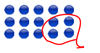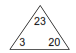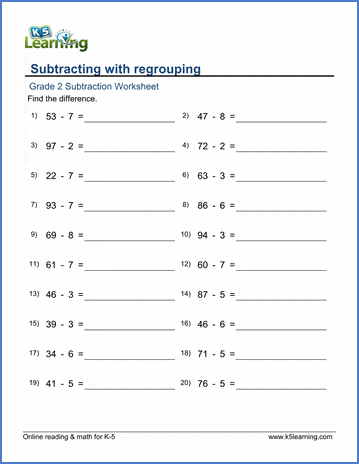## Basic subtraction worksheets

Our grade 2 subtraction worksheets provide the practice needed to master basic subtraction skills. They cover 2nd grade topics ranging from basic subtraction facts to subtracting in columns with regrouping.

## Mental subtraction

Subtraction sentences## Subtracting within 1-20

Subtract within 0-10 9 - 3 =
Subtract within 0-10, missing minuend or subtrahend __ - 5 = 2
Subtract within 10-19 18 - 11 =

## Subtracting a single digit number

Subtract 1-digit from a 2-digit number (no regrouping) 35 - 4 =
Subtract 1-digit from a whole ten 20 - 2 =
Subtract 1-digit from a 2-digit number 33 - 9 =
Subtract 1-digit from a 3-digit number 217 - 9 =

## Missing minuend / subtrahends

Subtract 1-digit from a 2-digit number; missing numbers (no regrouping) 66 - __ = 61
Subtract 1-digit from a 2-digit number, missing minuend or subtrahend 66 - __  = 58
Subtract 1-digit from a 3-digit number, missing minuend or subtrahend 103 - __ = 96

## Subtracting whole tens

Subtract whole tens from whole tens within 0-1,000 900 - 50 =
Subtract whole tens, missing minuend or subtrahend, within 0-1,000 __ - 70 = 230
Subtract whole tens from 2-digit numbers 36 - 30 =
Subtract whole tens from 3-digit numbers, no regrouping 271 - 50 =
Subtract whole tens from 3-digit numbers 159 - 60 =

## Subtracting whole hundreds

Subtract whole hundreds from 3-digit numbers 729 - 200 =
Subtract a 2-digit number from a whole hundred 700 - 77 =

Fact familiesAdd and subtract 3 single-digit numbers 7 + 1 - 4 =
Add and subtract 4 single-digit numbers 4 + 7 - 5 - 5 =

## Subtracting in columns

Subtract a 2-digit number from a 2-digit number (no borrowing) 96
- 54

Subtract two-digit numbers in columns - missing minuend or subtrahend 26
-
15
Subtract a 3-digit number from a 3-digit number (no borrowing) 798
- 123

Subtract 3-digit numbers in columns - missing minuend or subtrahend 543
-
210

## Subtracting in columns (with borrowing)

Subtract a 2-digit number from a 2-digit number with borrowing 96
-87

Subtract a 3-digit number from a 3-digit number with borrowing 421
- 388

Borrow over zeros 200
- 199

## Subtraction word problems

Simple subtraction word problems (1-2 digits)
Subtract 1-3 digit numbers
Mixed addition and subtraction within 20
Mixed addition and subtraction (2 digits)
More mixed addition and subtraction word problems